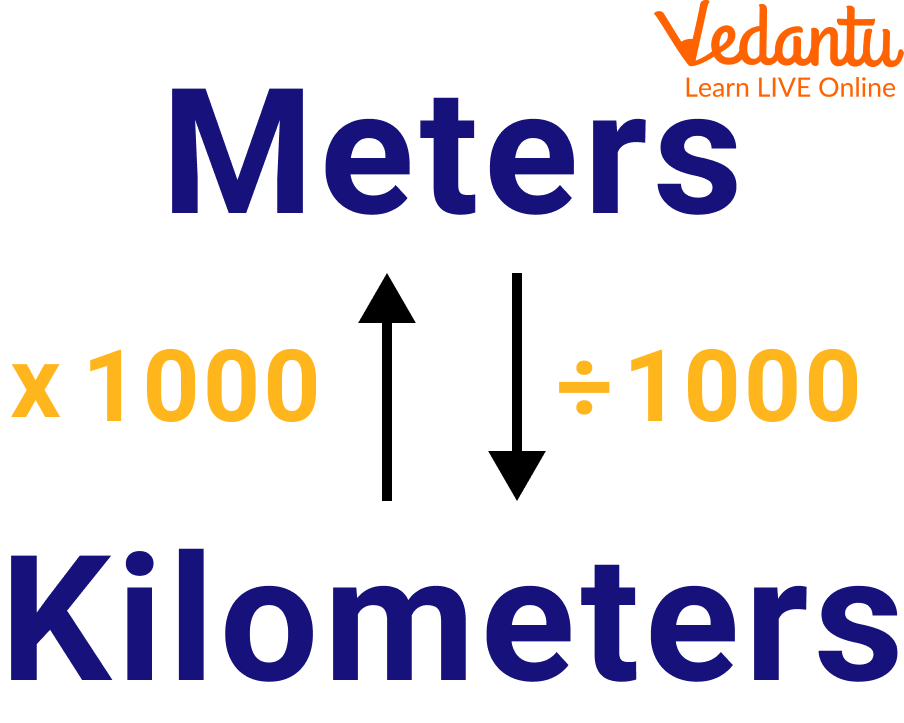Courses
Courses for Kids
Free study material
Free LIVE classes
More

# Learn How to Convert Metre Into Kilometre!LIVE
Join Vedantu’s FREE Mastercalss

## An Introduction to Metre to Kilometre Conversion

By providing the definition of metre and kilometre, we also get to know about converting metre into kilometre. Accordingly, in the International System of Units, a kilometre represents one mile (SI). The symbol for it is km. Km is typically used to describe a location's or a piece of land's distance. As 1 km = 1000 m, a metre can measure both length and distance. It is an SI unit with the symbol "m." The unit of measurement for length and distance is the metre. In this article, we will learn how to convert m to km.Symbols

## How to Convert m to km?Conversion of m to km

The SI unit of distance is the metre, as we all know. The units of measurement for length and distance are metres and kilometres. The metric system's unit of measurement for distance is the kilometre or 1,000 metres. One metre is, therefore, equal to one-thousandth of a kilometre. The symbol for a metre is m, while the symbol for a kilometre is km.

• To convert from m to km, we have to divide by 1000.

• To convert from km to m, we have to multiply by 1000.

## How Many Metres in a Kilometre?

A kilometre, often known as a km, is one thousand metres, or roughly $0.621$ miles, in length. It is the most popular unit for determining the distance between locations throughout most of the world. The fundamental unit of length in the metric system, upon which all other length units are built, is the metre, or metre. It is equivalent to $100 \mathrm{~cm}$, or roughly $39.37$ inches, or $\dfrac{1}{1000}$ th of a kilometre. Knowing the conversion factor allows us to quickly and easily convert 1000 metres to kilometres by multiplying $0.001$ by the number of metres we have, which is 1000.

$1000 \times 0.001=1 \mathrm{~km}$

As is common knowledge, a kilogramme is equal to 1000.

There are 1000 metres in a kilometre.

This implies that when converting 1000 metres to km, it makes up 1 kilometre.

Consequently, one kilometre is equal to 1000 metres.

## Metre to Km Conversion Chart

The metre to km conversion chart is given below:

 Metres Kilometres 1 m 0.001 Km 2 m 0.002 km 3 m 0.003 km 4 m 0.004 km 5 m 0.005 km 6 m 0.006 km 7 m 0.007 km 8 m 0.008 km 9 m 0.009 km 10 m 0.01 Km 20 m 0.02 Km 30 m 0.03 Km

## Solved Examples

Q1. 6000 metres to kilometres

Ans: 1000m = 1km

So, 6000 is divided by 1000

Quotient = 6

Remainder = 0

Q2. 10000 metres to kilometres

Ans: 10000m = 1km

So, 10000 is divided by 1000

Quotient = 10

Remainder = 0

Q3. 7856 m to km

Ans: 1000m = 1km

So, 7856 is divided by 1000

Quotient = 7

Remainder = 856

Thus, answer = 7 km 856 m (7000+856) or 7.856 km

## Practice Questions

Q1. 9999 m to km

Ans: 9 km 999 m

Q2. 1210 m to km

Ans: 1 km 210 m

Q3. State true or false:

Metre is the standard unit to measure the length.

Ans: True

## Summary

The process of converting metres into kilometres is simple and only requires a few steps. First, identify the number of metres you wish to convert. Second, divide that number by 1,000 to find the equivalent number of kilometres. Third, round off the answer to the nearest tenth if necessary. And that's it! You've now successfully converted metres into kilometres. Try doing a few more conversions on your own to practise. Once you have mastered this conversion, you will be able to easily calculate distances in both metric units. So next time someone asks you how far away something is, don't hesitate to give them an answer in either unit of measurement!

Last updated date: 28th Sep 2023
Total views: 90.9k
Views today: 1.90k

## FAQs on Learn How to Convert Metre Into Kilometre!

1. Is a yard or metre longer?

Both yards or metres are used to measure the distance or length of the two objects.  While comparing the difference between yards and metres, one metre is equal to 1.09 yards.

2. Is 100m equal to 1 km?

A kilometre is a unit of measurement that is equivalent to 1,000 metres because the Greek prefix kilo- signifies "one thousand" in English. Since 1,000 is a significant number, long distances are typically measured in kilometres. For instance, kilometres can be used to determine the distance between two points in a country. The term "kilometre" can be shortened, just like other words for units of measurement. Kilometres are denoted by the abbreviation km.

3. Why do they use km in India?

India first used British measurements, but eventually moved to metric or SI units. Therefore, the earlier generations are familiar with both miles and kilometres, while the latter generations are only familiar with kilometres. Today, the metric system is used for all official measures. Additionally, the metric system makes it simple to convert between units and to calculate magnitudes such as speed and velocity.